# 04-00 Deep_Neural_Network–线性模型的局限性

03章节的TensorFlow入门我们建立了一个线性的模型Wx+b，实现了针对图片训练的简单逻辑分类器。这个功能看起来不错，但是功能却是十分有限。我们需要引入非线性的元素来实现更多的功能。

# 线性模型中的参数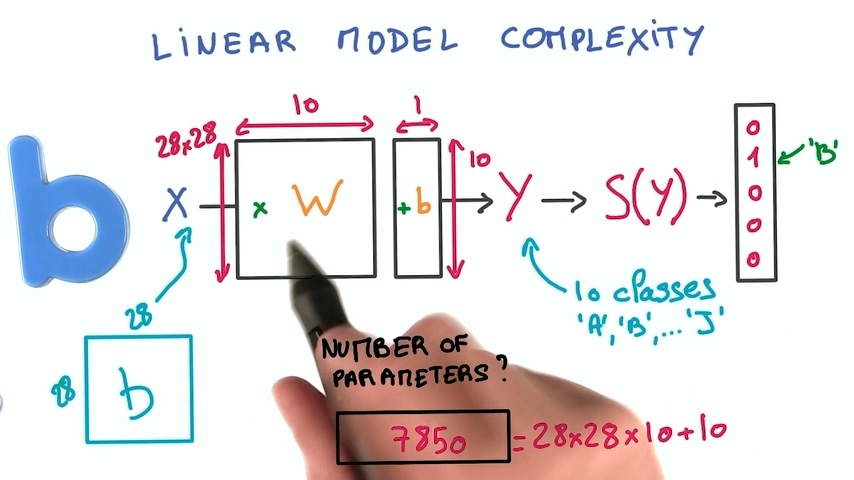# 线性模型的局限性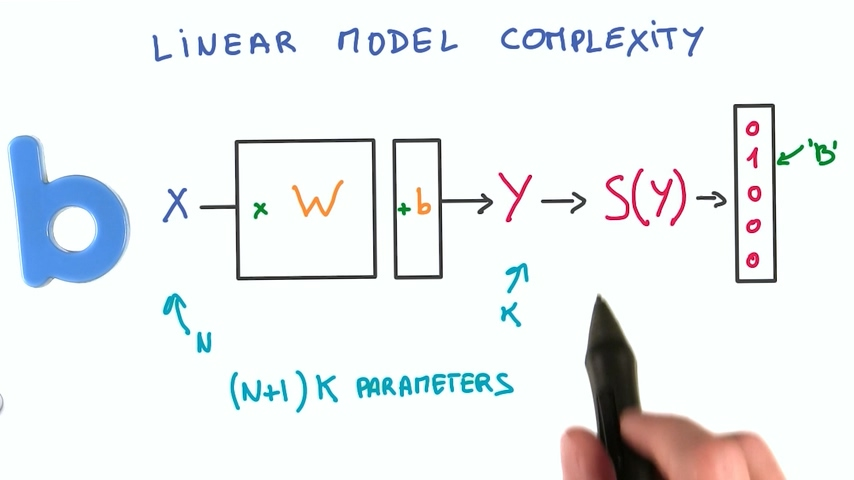– 关系式为两个输入相加，线性模型可以很好表示
– 关系式为两个输入相乘，线性模型无法表示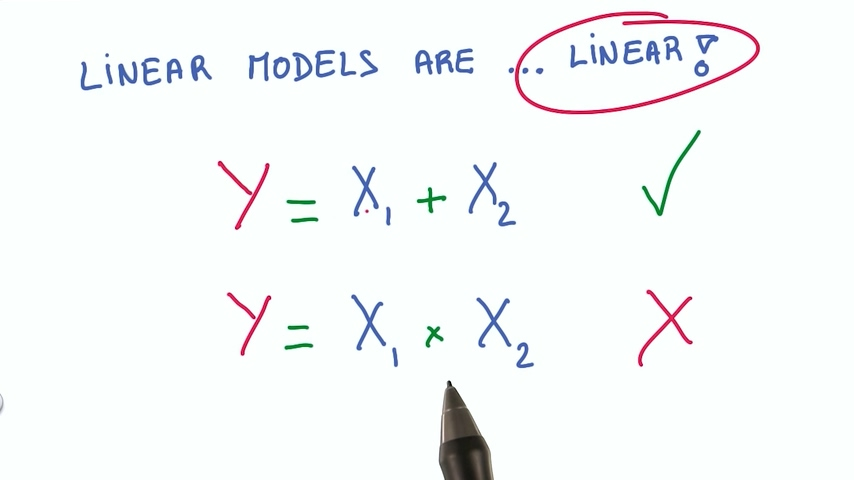– 线性模型效率非常高，它的计算就是矩阵乘法（wx+b），而矩阵乘法我们用GPU来算非常方便
– 线性模型是非常稳定的，输入值小幅度变化，输出值同样是小幅度变化
– 线性模型的导数也很稳定，线性模型的导数是常数，没有比常数更稳定的吧？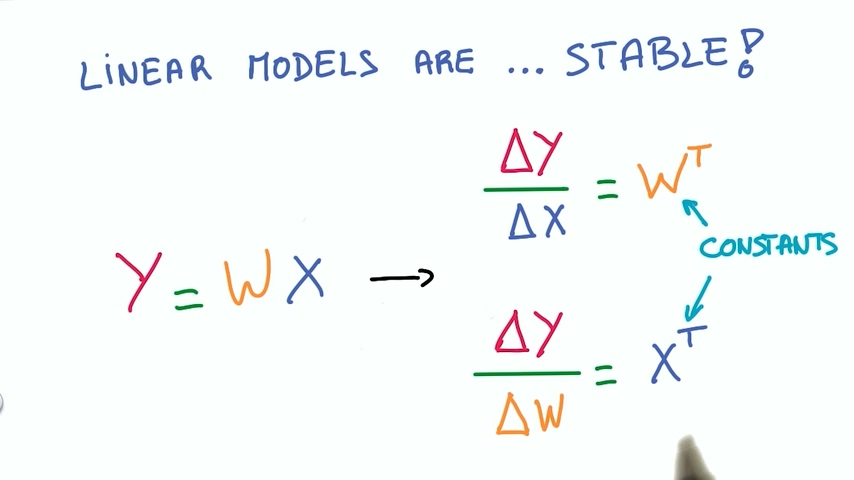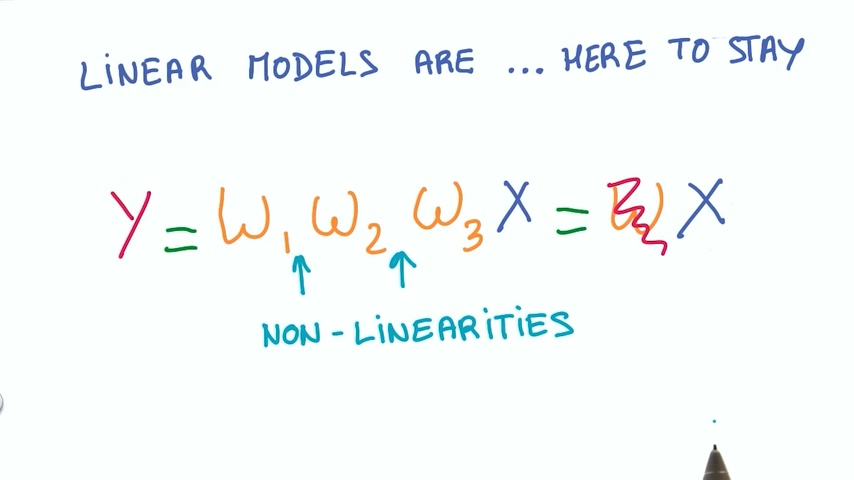# 最简单的非线性函数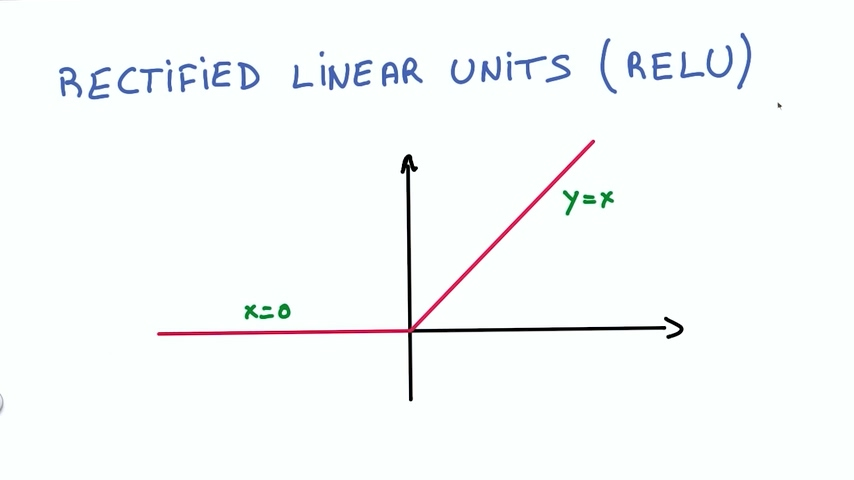ReLU也非常稳定，它的导数是两个常数。

# 应用ReLU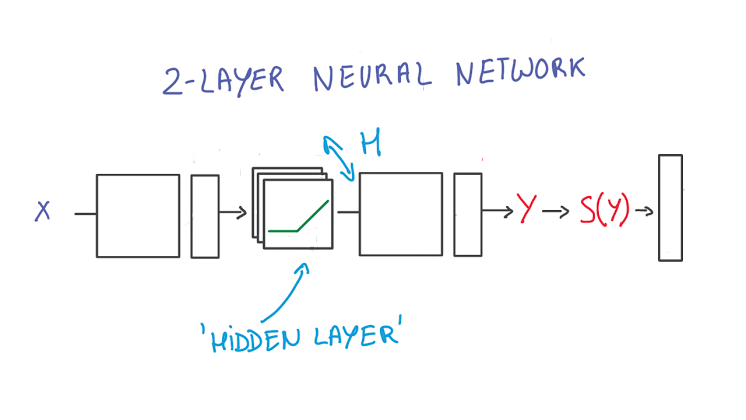# TensorFlow中的ReLU

``````# Hidden Layer with ReLU activation function
hidden_layer = tf.add(tf.matmul(features, hidden_weights), hidden_biases)
hidden_layer = tf.nn.relu(hidden_layer)

output = tf.add(tf.matmul(hidden_layer, output_weights), output_biases)
``````

ReLU其实是个激活函数，眼尖的同学肯定会想起我们之前学的sigmoid函数，我们在代码中比较一下这两个函数的不同

``````import tensorflow as tf

output = None
hidden_layer_weights = [
[0.1, 0.2, 0.4],
[0.4, 0.6, 0.6],
[0.5, 0.9, 0.1],
[0.8, 0.2, 0.8]]
out_weights = [
[0.1, 0.6],
[0.2, 0.1],
[0.7, 0.9]]

# Weights and biases
weights = [
tf.Variable(hidden_layer_weights),
tf.Variable(out_weights)]
biases = [
tf.Variable(tf.zeros(3)),
tf.Variable(tf.zeros(2))]

# Input
features = tf.Variable([[1.0, 2.0, 3.0, 4.0], [-1.0, -2.0, -3.0, -4.0], [11.0, 12.0, 13.0, 14.0]])

# TODO: Create Model
hidden_layer = tf.add(tf.matmul(features, weights), biases)
hidden_layer = tf.nn.relu(hidden_layer)
logits = tf.add(tf.matmul(hidden_layer, weights), biases)

# TODO: Print session results
with tf.Session() as sess:
sess.run(tf.global_variables_initializer())
print("relu result:")
print(sess.run(logits))

sigmoid_hidden= tf.nn.sigmoid(sigmoid_hidden)
sigmoid_logits = tf.add(tf.matmul(sigmoid_hidden, weights), biases)
with tf.Session() as sess:
sess.run(tf.global_variables_initializer())
print("Sigmoid result:")
print(sess.run(sigmoid_logits))

``````

``````relu result:
[[ 5.11      8.440001]
[ 0.        0.      ]
[24.010002 38.239998]]
Sigmoid result:
[[0.99391145 1.5915966 ]
[0.00608859 0.00840352]
[1.         1.6       ]]
``````

Github链接

# 更多文章请关注我的个人网站：

weiweizhao.com

0 回复

### 发表评论

Want to join the discussion?
Feel free to contribute!